UART Communication

Description:

• In UART communication, two UARTs communicate directly with each other.
• The transmitting UART converts parallel data from a controlling device like a CPU into serial form, transmits it in serial to the receiving UART, which then converts the serial data back into parallel data for the receiving device.
• Only two wires are needed to transmit data between two UARTs.
• Data flows from the Tx pin of the transmitting UART to the Rx pin of the receiving UART
• UARTs transmit data asynchronously, which means there is no clock signal to synchronize the output of bits from the transmitting UART to the sampling of bits by the receiving UART.
• Instead of a clock signal, the transmitting UART adds start and stop bits to the data packet being transferred.
• These bits define the beginning and end of the data packet so the receiving UART knows when to start reading the bits.
• When the receiving UART detects a start bit, it starts to read the incoming bits at a specific frequency known as the baud rate.
• Baud rate is a measure of the speed of data transfer, expressed in bits per second (bps).
• Both UARTs must operate at about the same baud rate.
• The baud rate between the transmitting and receiving UARTs can only differ by about 10% before the timing of bits gets too far off.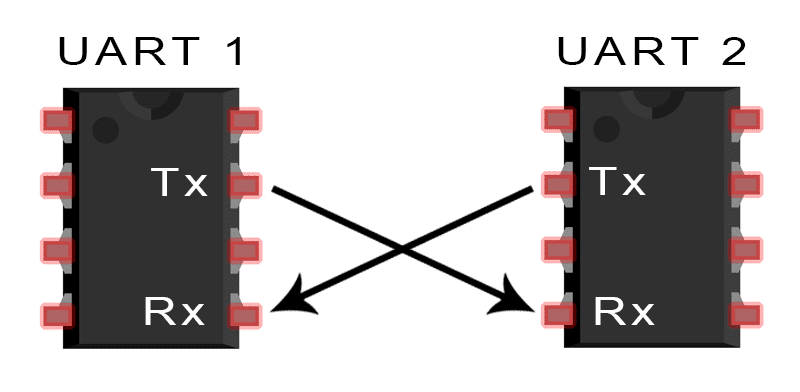Working Principle:

• The UART that is going to transmit data receives the data from a data bus.
• The data bus is used to send data to the UART by another device like a CPU, memory, or microcontroller.
• Data is transferred from the data bus to the transmitting UART in parallel form.
• After the transmitting UART gets the parallel data from the data bus, it adds a start bit, a parity bit, and a stop bit, creating the data packet. Next, the data packet is output serially, bit by bit at the Tx pin.
• The receiving UART reads the data packet bit by bit at its Rx pin.
• The receiving UART then converts the data back into parallel form and removes the start bit, parity bit, and stop bits.
• Finally, the receiving UART transfers the data packet in parallel to the data bus on the receiving end: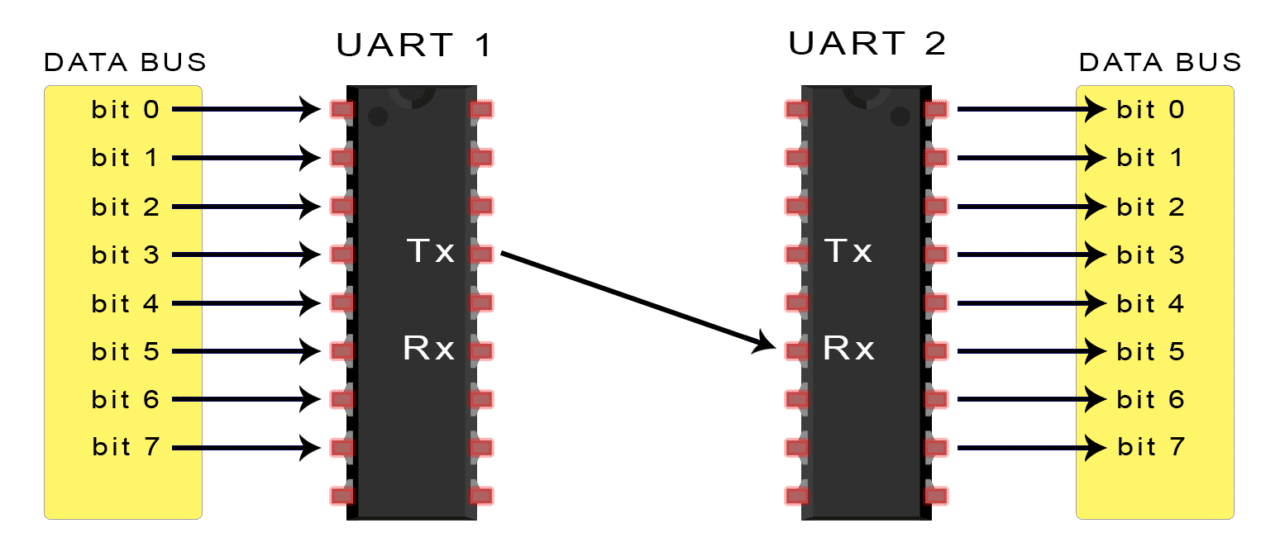UART transmitted data is organized into packets. Each packet contains 1 start bit, 5 to 9 data bits (depending on the UART), an optional parity bit, and 1 or 2 stop bits:

Start Bit

The UART data transmission line is normally held at a high voltage level when it’s not transmitting data. To start the transfer of data, the transmitting UART pulls the transmission line from high to low for one clock cycle. When the receiving UART detects the high to low voltage transition, it begins reading the bits in the data frame at the frequency of the baud rate.

Data Frame

The data frame contains the actual data being transferred. It can be 5 bits up to 8 bits long if a parity bit is used. If no parity bit is used, the data frame can be 9 bits long. In most cases, the data is sent with the least significant bit first.

Parity

Parity describes the evenness or oddness of a number. The parity bit is a way for the receiving UART to tell if any data has changed during transmission. Bits can be changed by electromagnetic radiation, mismatched baud rates, or long distance data transfers. After the receiving UART reads the data frame, it counts the number of bits with a value of 1 and checks if the total is an even or odd number. If the parity bit is a 0 (even parity), the 1 bits in the data frame should total to an even number. If the parity bit is a 1 (odd parity), the 1 bits in the data frame should total to an odd number. When the parity bit matches the data, the UART knows that the transmission was free of errors. But if the parity bit is a 0, and the total is odd; or the parity bit is a 1, and the total is even, the UART knows that bits in the data frame have changed.

### Stop Bits

To signal the end of the data packet, the sending UART drives the data transmission line from a low voltage to a high voltage for at least two bit durations.

## Steps of UART Transmission

1. The transmitting UART receives data in parallel from the data bus: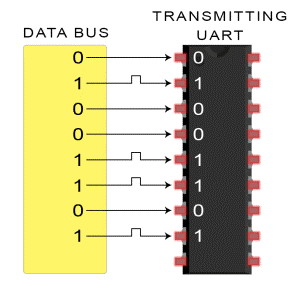1. The transmitting UART adds the start bit, parity bit, and the stop bit(s) to the data frame: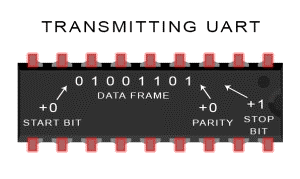3. The entire packet is sent serially from the transmitting UART to the receiving UART. The receiving UART samples the data line at the pre-configured baud rate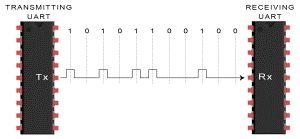4.  The receiving UART discards the start bit, parity bit, and stop bit from the data frame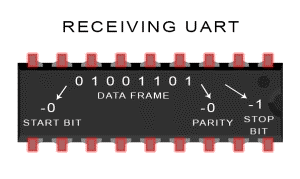1. The receiving UART converts the serial data back into parallel and transfers it to the data bus on the receiving end: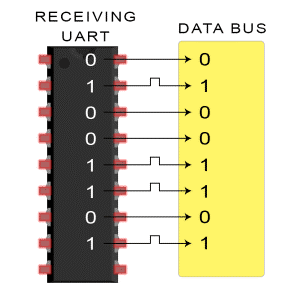WhatsApp us